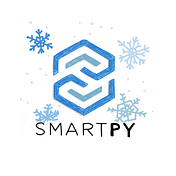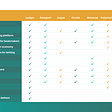# Change of Syntax

## Local Variables

• `sp.newLocal` is renammed `sp.local` (this is done automatically)
• if `loc` is a local variables, its value is set and accessed to by using `loc.value`. For example, we now write `loc.value = 3 + 2 * loc.value`.
`    @sp.entry_point    def squareRoot(self, x):        sp.verify(x >= 0)        y = sp.local('y', x)        sp.while y.value * y.value > x:            y.value = (x // y.value + y.value) // 2        sp.verify((y.value * y.value <= x)                   & (x < (y.value + 1) * (y.value + 1)))        self.data.value = y.value`

`c1 = MyContract(12, 123)    scenario += c1    # And call some of its entry points    scenario += c1.myEntryPoint(12)    scenario += c1.myEntryPoint(13)    scenario += c1.myEntryPoint(14)    scenario += c1.myEntryPoint(50)    scenario += c1.myEntryPoint(50)    # this is expected to fail    scenario += c1.myEntryPoint(50).run(valid = False)    # Finally, we check its final storage    scenario.verify(c1.data.myParameter1 == 151)`

## Test Accounts

`  admin = sp.test_account("Administrator")  alice = sp.test_account("Alice")  bob   = sp.test_account("Robert")`

## Showing Expressions

`scenario.show(expression, html = True, stripStrings = False)scenario.show(c1.data)scenario.show(c1.data.l)`

## Computing Expressions

`x = scenario.compute(c1.data.myParameter1 * 12)`

## Testing Equality

`# Verifying a boolean expression that happens to be an equalityscenario.verify(c1.data.myParameter == 51)# Verifying an equalityscenario.verify_equal(c1.data.myList == [2, 3, 5, 7])`

# How To Contribute

• Providing documentation
• Writing templates
• Reporting bugs and asking for improvements

--

--

--

## More from SmartPy.io

An intuitive and effective smart contracts language and development platform for Tezos. In Python.

Love podcasts or audiobooks? Learn on the go with our new app.## SmartPy.io

An intuitive and effective smart contracts language and development platform for Tezos. In Python.

## Introduction to Blockchain and it’s Use Cases## The Evolution Of Blockchain Technology Over These Years — A Remarkable Achievement!## Two Months In and Just Getting Started## The Ultimate guide to understanding Blockchain technology for non-techies.